A Framework for Multi-Factor Fundamental Equity Risk Model | OMICS International
Journal of Business & Financial Affairs

# A Framework for Multi-Factor Fundamental Equity Risk Model

Ashish Garg*

*Corresponding Author:
Ashish Garg, PhD
Tel: (310) 954-6922
E-mail: [email protected]

Received Date: March 28, 2017; Accepted Date: March 29, 2017; Published Date: March 31, 2017

Citation: Garg A (2017) A Framework for Multi-Factor Fundamental Equity Risk Model. J Bus Fin Aff 6: e149. doi: 10.4172/2167-0234.1000e149

Copyright: © 2017 Garg A. This is an open-access article distributed under the terms of the Creative Commons Attribution License, which permits unrestricted use, distribution, and reproduction in any medium, provided the original author and source are credited.

Visit for more related articles at Journal of Business & Financial Affairs

The concepts of equity risk have evolved from calculation of simple standard deviation of past returns to advanced multi-factor models today. The complex portfolios, today, that contain multiple securities to advantage from diversification principle and from different risk return profile, render the traditional practices of risk modeling and calculations inept in measuring the portfolio risk exposure or improving the portfolio’s risk-adjusted performance for portfolio managers. Multifactor models allow for more thorough understanding of portfolio’s risk exposures to implicit and explicit variables . They are proving to be useful not only for risk management purposes, but also in portfolio performance attribution and providing basis for improved portfolio construction.

There are three types of factor models that are widely used, namely, the first is the statistical factor models, second is explicit factors model, and third is explicit/implicit factors model that take statistical factor modeling elements also into account. There are mainly three guiding principles of these models: first - portfolio returns are primarily driven by set of common factors, second – portfolio risk comes from volatility and correlation among these factors, third – there is idiosyncratic risk that is not captured by the common factors. Next, each of the three types of modeling approaches is discussed. The explicit/implicit factors model framework discussion is further expanded by taking an example of S&P500 index .

The statistical factor models generally use principal component modeling approach where both factor returns and factor exposures are determined from the factor returns. Principal Component Analysis (PCA) is a very powerful mathematical technique that reduces the dimensionality of complex problems by identifying the latent factors. For example, a portfolio has 500 stocks in it. To analyze the risk of this portfolio, one would require a covariance matrix of 500 x 500. The problem becomes very unwieldy not only from computational power standpoint but also from developing a meaningful conclusion from the analysis . The PCA can reduce this problem into highly reduced number of factors say 20 that can describe the dynamics of risk and return of all the 500 stocks in the portfolio.

The explicit factor models make use of set of explicit factors including macro-economic factors, industry/sector performance, accounting metrics. In these models, the factor returns are specified and factor exposures are determined by regression of asset/stock returns on factor returns. The limitation of this approach is that not all the factors are observable. Even is sometimes they are observable, their data quality is not robust enough to be able to be used in regression analysis.

The explicit/implicit factor models make use of both implicit and explicit set of variables. The explicit factors include the factors for which metrics/data is directly available like mentioned above macroeconomic factors, industry/sector performance accounting metrics. The implicit factors are the ones which are derived by specifying the factor exposures and then, the factor returns are estimated by regression of asset returns on factor exposures.

The remainder of the paper discusses a framework of multifactor fundamental equity risk model. First, there is a discussion of the potential factor structure that includes industry, fundamental, and technical factors, followed by a discussion on factor risk model specification, then the estimation steps for implicit factors return, and finally the volatility model specification which could be based on EWMA or GARCH approach, but in this framework an example of EWMA is illustrated.

#### Factor Structure

Industry factors

The Global Industry Classification Standard (GICS) is globally accepted industry taxonomy . There are 4 levels of classification under it: 11 sectors, 24 industry groups, 68 industries, and 157 sub industries. The first or second levels tend to serve as enough comprehensive classifications for risk model purposes. The table below shows the 10 sectors that has traditionally existed in the GICS level 1 and “Real Estate” has been recently added (Table 1).

Energy Health Care
Consumer Discretion Telecommunication
Materials Utilities
Industrial Consumer Staples
Information Technology Finance

Table 1: GICS level 1 classification.

Fundamental factors

These consist of factors related to financial health of the company like value, size, growth, leverage, profitability and dividend yield. These factors can be usually derived from the company’s balance sheet and income statements.

a) Value: It refers to the set of stocks that trade at a lower price relative to its fundamentals. Some characteristics used to construct value factor as this is not directly observable factor are dividend yield, price-to-book ratio, and price-to-earnings ratio.

b) Size: It refers to the set of stocks that have high market capitalization.

c) Growth: It refers to the set of stocks or companies whose earnings are expected to grow at a higher rate than market.

d) Leverage: It refers to debt servicing capabilities of the companies.

e) Profitability: It refers to balance sheet item such as income, book value, profitability ratios.

f) Dividend yield: It represents the cash flow the company gives back to its investors from its total earnings.

The below Table 2 explains the potential methodology, that can be used to estimate each of the fundamental factors listed above.

Value Size
Descriptors used were Descriptors used were
1. Back to price (B/P) 1. Log (Market Cap)
2. Earnings to price (E/P) 2. Log (Sales)
3. Log (Total Assets)
Value=0.5 (B/P+E/P) Size=0.33 (MktCap+Sales+Total Assets)
Growth Leverage
Descriptors used were Descriptors used were
1. Total Asset Growth (TAG) 1. Total Debt
2. Earnings Growth (TEG) in   two years 2. Firm Value
Growth=0.5 (TAG+TEG) Leverage =Total Debt / Firm Value
Profitability Dividend Yield
Descriptor used Descriptor used
1. Income 1. Dividends
2. Book Value 2. Price
Profitability =Income / Book Value Dividend Yield =Dividend / Price

Table 2: Methodology to estimate the fundamental factors.

Technical factors

These consist of factors related to the performance of the stock in the market. These factors are derived from the time series of the stock prices, returns and trading volumes .

Table 3 explains the potential methodology, which can be used to estimate the technical factors.

 Momentum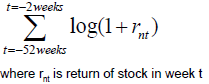Liquidity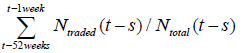Volatility Calculated as volatility in returns over past 26 weeks

Table 3: Methodology to estimate the technical factors.

#### Factor Risk Model

Once the factor structure is established , the next step involves model specification for return generating process from the factors which can be laid out as: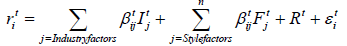Where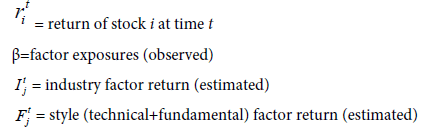Rt=residual systematic movement in stock returns

#### Factors Estimation Modeling Steps

In the above framework, the modeling process to estimate implicit factor returns involves 3 steps

Estimation of industry factors returns

For each cross-sectional data univariate regression can be done as follows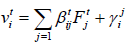Where the loading, beta, can be assumed to be 1 for the industry that stock belongs to and zero for all the other industries.

Estimation of fundamental and technical factor returns

The residual returns from the above regression can be used to regress factor loadings in this step. This gives factor return for a given period as follows

<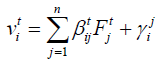/p>

Estimation of idiosyncratic returns

Finally, the idiosyncratic returns can be calculated as average of residual returns from the second step as follows: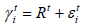#### Volatility Model

EWMA volatility model is based on the concept of exponentially weighted moving average. It tend to provide the better estimate of forecasted volatility than simple variance as it puts more weight on recent return/volatilities and that weight reduces in the exponent manner for old observations. It uses the smoothing parameter lambda. RiskMetricsTM, a financial risk management company, uses a value of 0.94 for lambda, and has been adopted as industry practice. The portfolio risk can be calculated as follows using the EWMA framework [7,8].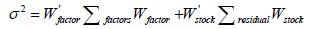Where covariance matrix is calculated by first applying EWMA on return series as follows: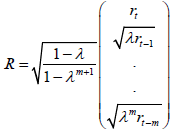Where

R=New time series a factor return

R=Initial time series of the same factor return

#### References

Select your language of interest to view the total content in your interested language

### Article Usage

• Total views: 3011
• [From(publication date):
March-2017 - Feb 21, 2020]
• Breakdown by view type
• HTML page views : 2754Can't read the image? click here to refresh